International Journal of Electrical Components and Energy Conversion
Volume 2, Issue 3, June 2016, Pages: 15-20

A Dual-mode Control of a Multi-coil Electromechanical Converter Based on an Improved Bacterial Foraging Algorithm

Gong Zhang1, 2, *, Zheng Xu1, Weijun Wang1, 2, Zhichen Hou1, Xing Gu1, Songsong Liang1,
Ning Lin1, Yunpeng Guo1

1Guangzhou Institute of Advanced Technology, Chinese Academy of Science, Guangzhou, China

2Shenzhen Institute of Advanced Technology, Shenzhen, China

Email address: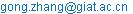(Gong Zhang)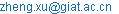(Zheng Xu)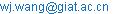(Weijun Wang)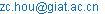(Zhichen Hou)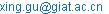(Xing Gu)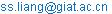(Songsong Liang)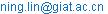(Ning Lin)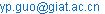(Yunpeng Guo)

*Corresponding author

To cite this article:

Gong Zhang, Zheng Xu, Weijun Wang, Zhichen Hou, Xing Gu, Songsong Liang, Ning Lin, Yunpeng Guo. A Dual-mode Control of a Multi-coil Electromechanical Converter Based on an Improved Bacterial Foraging Algorithm. International Journal of Electrical Components and Energy Conversion. Vol. 2, No. 3, 2016, pp. 15-20. doi: 10.11648/j.ijecec.20160203.11

Received: August 4, 2016; Accepted: August 13, 2016; Published: September 13, 2016

Abstract: The objective of this research is to improve the response performance of an electro-mechanical converter (EMC), a new design of EMC provided with the bobbin of triple coils is proposed in this study. A mathematic model and a solution of searching time optimum using an improved Bacterial Foraging Algorithm (BFA) are developed, and a dual-mode controller with Bang-Bang & PI based on the proposed method is designed. Analysis and simulation results show promising effects that the response time of the triple coil EMC driven by dual-mode controller is reduced from 8.5ms to 2.5ms compared to the traditional single coil EMC, and the ringing and overshoot are below 5%. The designed control technology can realize high response performance and display good prospect of development.

Keywords: Electromechanical Converter (EMC), Triple Coil, Bacterial Foraging Algorithm (BFA), Time Optimal Control, Bang-Bang Control

Contents

1. Introduction

An electromechanical converter (EMC) is regarded as the most widely employed linear motion mechanism in various industry driving fields, so that electromagnetic energy can be converted to mechanical energy reciprocating linear motion continuously and proportionately. At present, a moving coil type of EMC has been drawing widely attention because of high linearity and small hysteresis. Tanaka  pointed out the generated electromagnetic force is about 2.5 times higher than the others with the same size.

Step input is very common in automatic control applications. However, the optimal performance of the EMC is often compromised when driven by a conventional PID controller, since quickness, robustness and overshoot are contradictory in a PID controller [2-4]. K. A  built a third-order model that includes the inductance of the motor coil for Bang-Bang control with time optimal performance, and developed universal curves which enable designers to choose appropriate actuator performance parameters to fulfill different design objectives. Khandaker  and his group proposed a new control strategy based on applying high and low voltages pulse width modulated (PWM) power for transient and steady period, respectively, and achieved the conflicting requirements of very fast transition time and low contact velocities simultaneously. Mosca  applied the Affordable Predictive Control to the position servo control system in HDD, and the performance of good tracking, anti-jamming, robustness is provided.

However, the research above are not sufficient for searching a time optimal solution, some parameters are restricted to keep the model at overdamping, so that the underdamping state is not taken into consideration, and some models are simplified to avoid cross feedback.

The aim of this study is to improve those deficiencies above, and a dual-mode controller with Bang-Bang & PI based on Bacterial Foraging Algorithm (BFA) is designed to obtain a time optimal control without losing robustness.

2. Structure and Mathematical Model

A schematic of an EMC designed in this study is detailed in Figure 1, which consists of permanent magnetic (PM), coils, bobbin, iron core, covers, output shaft, connector, etc. Several blocks of magnetic are fixed on the inner face of the right cover. An iron core is fixed in the center of the right cover by a screw. The coils are wrapped on the bobbin. The Bobbin which can move axially in the air gap formed between the PM and the iron core is connected to a guide pin and an output shaft. Input voltage signal is provided to coils after being amplified. Thus, the bobbin and the output shaft connected to the carrying currents coils and will move under the effect of Ampere force.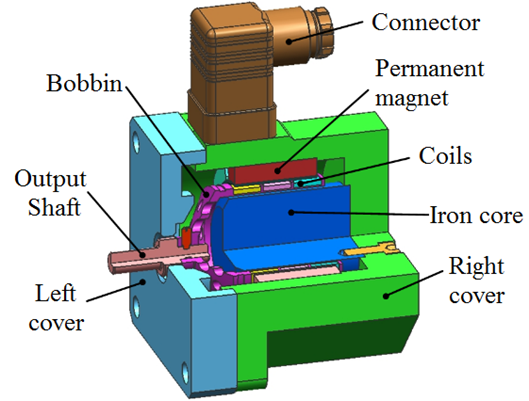Fig. 1. Schematic of the proposed EMC.

Figure 2 reveals the control principle of EMC. The feedback signal is generated by a position sensor on the output shaft. The controller receives input signal and feedback signal. After comparing the difference between them, the controller output driving signal to eliminate the error. Obviously, a closed-loop control system is proposed and displays high accuracy.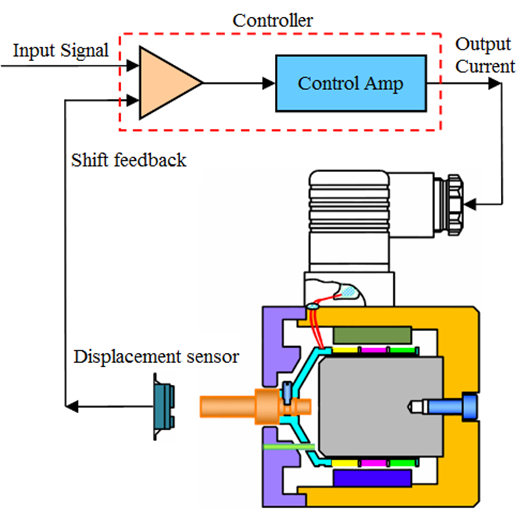Fig. 2. Control principle of EMC.

Figure 3(a) and 3(b) compare two different bobbins of EMC with single coil and triple coils, respectively, which both provides with the same basic dimensions but the outer face of the triple coil type is divided into three independent sections for wrapping wire. Each of the three coils exhibits lower impendence than the single one, which will generate lager current under the same supply voltage. In addition, the triple coils are energized independent, which allows more flexible control strategy and different current between them.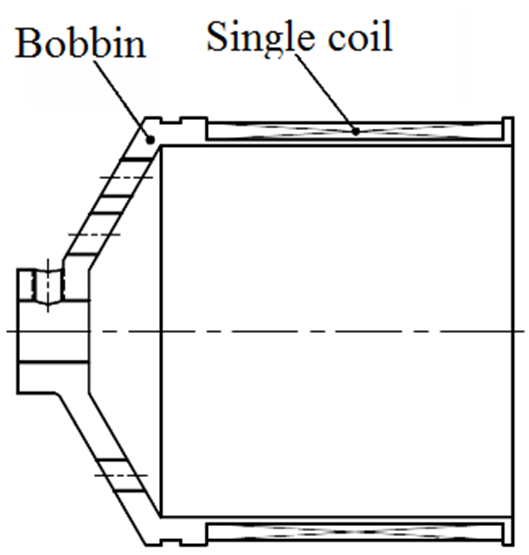(a) Single coil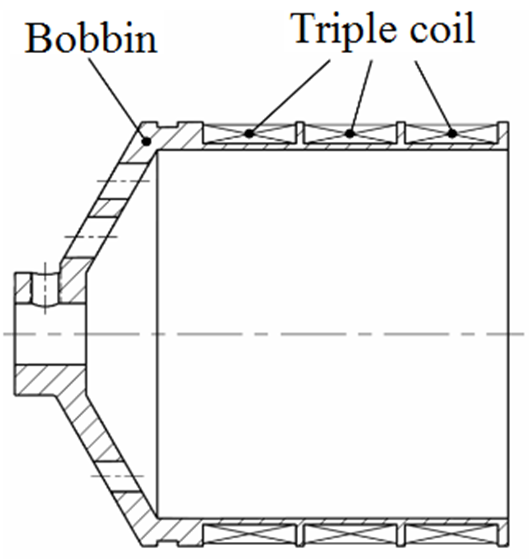(b) Triple Coil

Fig. 3. Two different bobbins of EMC.

The output force of the EMC proposed above is given by Ampere force equation :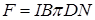(1)

Where I is coil current, B is magnetic strength, D is average diameter of the coils, N is turns of the coils.

For a certain EMC with known dimension and material, the factor BπDN is a constant which can be defined as the transducer constant Ke.

Considering the load connected to the EMC, equations can be given out. The equations of the single coil EMC is: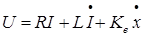(2)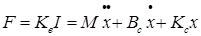(3)

Where U is supply voltage, R is resistance of the coil, L is inductance of the coil, Ke is transducer constant, M is total mass of EMC and load, Bc is damping coefficient, Kc is elastic constant, x is output displacement.

The equations of the triple coil EMC is: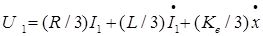(4)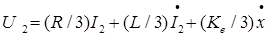(5)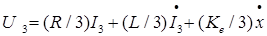(6)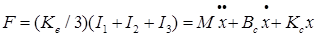(7)

Where Ui (i=1, 2, 3) is voltage applied to each coil, respectively, Ii (i=1, 2, 3) is current of each coil, respectively

It can be considered briefly that the impedance and transducer constant of each coil in triple coils are one third of the single coil, and will work at maximum power while paralleled , and the equations (4) ~ (7) can be simplified as: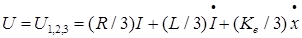(8)(9)

where I=I1=I2=I3

Comparing equation (2) and (8), it is obvious that an EMC with three paralleled coils is equal to a single coil EMC with one third impedance and the same transducer constant, and the goal of lowing impedance is achieved according. Let mechanical quantities to be as follows: x1=x (displacement), x2=x’ (velocity), x3=x’’ (acceleration). Combining (8) and (9), the system can be expressed as: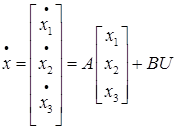(10)

where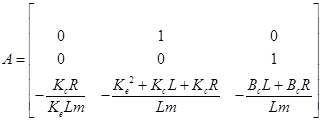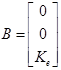3. Dual-mode Controller

The equation matrix (10) matches the standard format of a completely controllable single input system. According to the Pontryagin’s Minimum Principle, the time-optimal control U(t) is a Bang-Bang type and the solution is unique. The maximum switching times are twice, which is half less than the order of the system [10,11].

However, the actual EMC system exhibits some uncertain character, such as the error of parameter, the changes in load, the non-differentiable part caused by friction, and some un-modeled dynamics. Bang-Bang control cannot deal with those influence, thus other method is needed to improve stability and robustness [13-15].

To solve the contradiction, we proposed a Dual-mode controller combined PI and time-optimal bang-Bang control together. In the start-up phase, the error is relatively large, the Bang-Bang control is applied, and three coils are paralleled for a fastest speed. While in the ending phase, the error is much smaller, thus, the closed-loop PI control is employed, and only one coil is working to guarantee the convergence of the desired final state. Figure 4 details a dual-mode control flow chart of EMC, the decision-making logic select either bang-bang mode or PI mode, the output signal is amplified by H-Bridge for driving the EMC, and the feedback signal is necessary as well.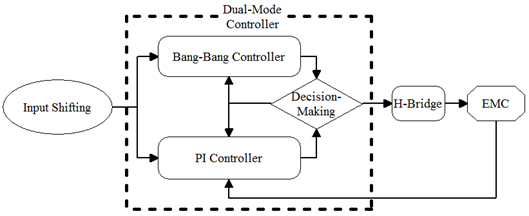Fig. 4. A dual-mode control flow chart of EMC.

3.1. Improved BFA for Time-Optimal Solution

According the analysis above, the problem is to search switching times to keep final time shortest under the constraining of displacement, velocity and acceleration. A Bacterial Foraging Algorithm (BFA) is introduced to search the solution. BFA is a bionic random search intelligent algorithm proposed by Passino  in 2002 provided with the advantages of parallel searching, easily escaping a local minimum.

Instead of optimizing the switching time directly, we define the duration of each input to be optimized as: t1, t2, t3, which means: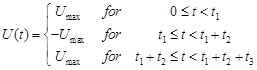The processes of BFA is briefly described as follows:

1)  Chemotaxis: Suppose Pi (j, k, l) represent the ith bacterium at jth chemotactic, kth reproductive and lth elimination-dispersal step. C(i) represents the step length and V(j) represents the random direction vector. Thus, in each chemotactic step, the movement of the ith bacterium can be represented as: Pi(i+1, j, k)= Pi(i, j, k) + C(i)V(j).

2)  Reproduction: After Nc times of chemotaxis process, bacteria will reproduce. All bacteria are sorted in a reverse order according to their health value. In this step, only the healthiest half of the population survive and splits into two identical ones, which are then placed in the same locations. Thus, the population of bacteria keeps constant.

Elimination-Dispersion: This process improves the diversity of bacteria to prevent being trapped into the local optima. This will move some bacteria to another position according to a preset probability p.

Through the description, the fixed step length would result in a contradictory between speed and accuracy. According to the research before, the difference between t1, t2, t3 can be as large as dozens of times [5,13]. In this way, the step length will be shorten for every finished reproduction process.

As the duration is either too short or too long compared to an optimal solution, we let the bacterium whose health value is decreasing turn to the opposite direction to prevent premature convergence. The improved chemotaxis process is presented in Figure 5.

In this case, since the optimizing is under the constraint of displacement(x1), velocity(x2) and acceleration(x3), penalty function method is easy-to-use to deal with the constraint . The health value in BFA can be expressed as: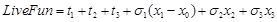(11)

where t1+t2+t3 is the performance index to be optimized, x0 is input displacement, σi (i=1, 2, 3) is penalty factor.

When penalty factor σi→∞ (i=1, 2, 3), the performance index converge to the optimum. Specifying σi require experiences and times of attempt, since if σi is too small, the constraint is too weak for convergence, if σi is too large, the solution will converge to a local optima. In this situation, x1 is the most important index, x2 is less important and x3 is the least. We therefore apply σ1=10, σ2=0.1, σ3=1×10-5 in this study.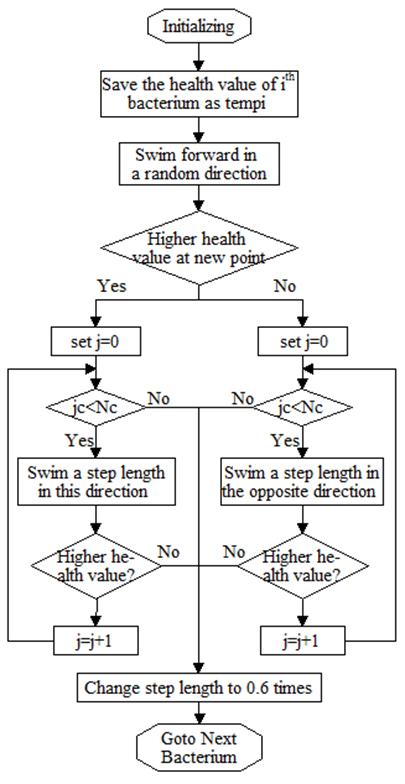Fig. 5. Improved Chemotaxis process of BFA.

3.2. Closed-Loop PI Controller

The PI controller is most widely control method in various applications, which includes a closed-loop PI displacement controller and a hysteresis comparator to regulate coil current. As this PI displacement controller is proposed to eliminate small displacement error, more aggressive parameters are acceptable, especially the "I" can be very large. In this study, we set: P=3×104, I=2×106.

4. Simulation and Discussion

Using software Matlab 2015 (MathWorks, Inc. USA) for control analysis to search the optimal solution. The analysis conditions are as follows: supply voltage Umax = 12V, start status x1=0, x2=0, x3=0, end status x1=1(mm), x2=0, x3=0, and other parameters are compared in Table 1. The calculation results are: t1=1.640ms, t2=0.341ms, t3=0.123ms, which can be also expressed as: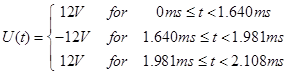Figure 6 gives the simulation results of response of triple coil EMC at step input of 1mm, it is obviously that all three mechanical quantities are settled to the required values.

Table 1. Parameter of EMC.

 Item Single coil Triple coil (for each) Mass M 0.2kg 0.2kg Resistance R 3.1Ω 1.03Ω Inductance L 0.5mH 0.167mH Supply voltage U 12V 12V Transducer constant Ke 31.25N·A-1 31.25N·A-1 Damping coefficient Bc 200 200 Elastic constant Kc 1940 1940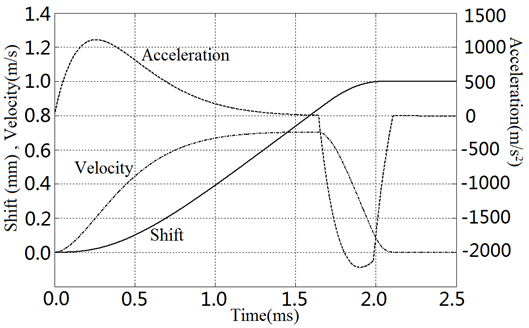Fig. 6. Response of triple coil EMC at step input of 1mm.

To illustrate a more detailed character of the triple coil EMC system, step displacement input from 0.2mm to 2.0mm with step length of 0.2mm are calculated, while the velocity and acceleration at the end and start point are 0, which is x1=0.2mm, 0.3mm…2.0mm, x2=0, x3=0. Figure 7 depicts the curves of displacement to excitation, the solid curve shows the total time cost, and the other three curves with marks indicate the duration of three excitation period, respectively. As detailed in Figure 7, the three-order model of EMC system exhibit saturation characteristics.

The comparison between the triple coil EMC with dual-mode controller and the general single coil EMC with PI controller is proposed in this study. The start status is x1=0mm, x2=0, x3=0 and the end status is x1=1.0mm, x2=0, x3=0. Other parameters are listed in Table 1. In addition, 5N of Coulomb friction is added to make it closer to reality.

Figure 8 represents the curves of response of two different EMCs at step input of 1mm. Curve "1" is the output displacement of triple coil EMC while curve "2" is the output displacement of single coil EMC. The triple coil EMC system settled to 1mm in 2.5ms with slightly overshoot and ringing. However, the single coil EMC system settled to 1mm after 8.5ms with relatively large overshoot and ringing, and the ringing and overshoot are below 5%.

The reason is that during the large error phase, the dual-mode controller is provided with the Bang-Bang type time-optimal solution so that the EMC can transfer to end status quickly, while during the small error phase, the PI part of the dual-mode controller eliminate the error result from Coulomb friction, which results in good robustness and ideal accuracy. The traditional PI controller possesses the contradiction between rapidity and stability so that the response is relatively slow to avoid large overshoot and ringing.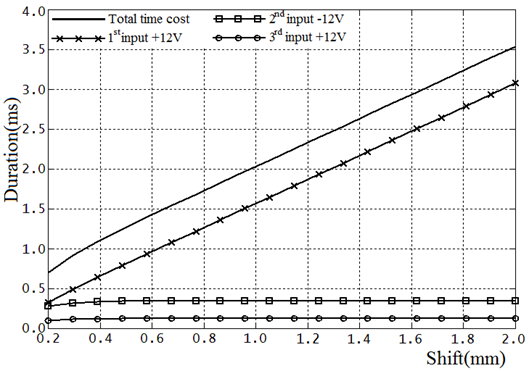Fig. 7. Curves of displacement to excitation.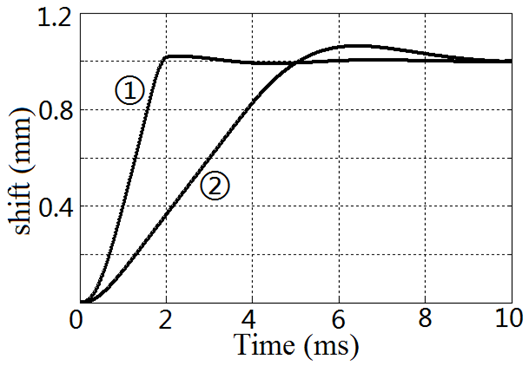Fig. 8. Response of two different EMCs at step input of 1mm.

5. Conclusion

A new design of EMC provided with triple coils is proposed in this study. A model and a solution of searching time optimum using an improved Bacterial Foraging Algorithm (BFA) are developed, and a dual-mode controller with Bang-Bang & PI based on the proposed method is designed. Analysis and simulation results show promising effects that the response time of the triple coil EMC driven by dual-mode controller is 2.5ms, 70% faster than the traditional single coil EMC system, and the ringing and overshoot are below 5%. As evident in this study, the designed control technology can realize high response performance and display good prospect of development.

Acknowledgments

This research is supported by the National Natural Science Foundation of China (Grant No. 51307170), the Shenzhen City Basic Research Projects of China (Grant No. JCYJ20140901003939032), and the Guangzhou City Scientific Research Projects of China (Grant No. 201607010041). The authors gratefully acknowledge the help of Guangzhou Institute of Advanced Technology, Chinese Academy of Sciences, and Shenzhen Institute of Advanced Technology.

References

1. H. Tanaka, "Progress of Electro-Magnetic Proportional Solenoids," Journal of the Japan Hydraulics & Pneumatics Society, vol. 31, no. 3, pp. 200-207, 2000
2. G. Zhang, J. Huang, X. S. Chen and R. X. Du, "Three-dimension magnetic field analysis of an electromechanical converter," Fifth Asia International Symposium on Mechatronics, Guilin, 2015, pp. 1-5.
3. G. Zhang, J. M Liang, C. M. Jiang, Z. P. Zhou, X. S. Chen and L. Y. Yu, "Computational fluid dynamics analysis of the air damping for an electromechanical converter," Lecture Notes in Electrical Engineering, 2015, vol. 373, pp. 907-914.
4. S. Zhao and K. K Tan, "Adaptive feedforward compensation of force ripples in linear motors," Control Engineering Practice, vol. 13, no. 9, pp. 1081-1092, Sep. 2005.
5. K. Ananthanarayanan, "Third-order theory and bang-bang control of voice coil actuators," IEEE Transactions on Magnetics, vol. 18, no. 3, pp. 888-892, May 1982
6. M. F. Khandaker, H. Hong and L. Rodrigues, "Modeling and controller design for a voice coil actuated engine valve," Proceedings of the 2005 IEEE Conference on Control Applications, Toronto, Ont., 2005, pp. 1234-1239.
7. E. Mosca, M. Baldini and P. Tesi, "Affordable predictive control of input-saturated plants and its use in hard disk drives," IFAC Proceedings Volumes, Vol. 40, no. 1, pp. 262-267, 2007.
8. H. Guo, D. Wang and J. Xu, "Research on a high-frequency response direct drive valve system based on voice coil motor," IEEE Transactions on Power Electronics, vol. 28, no. 5, pp. 2483-2492, May 2013.
9. L. W. Luo, G. Zhang, J. M. Liang, Z. K. Peng and X. S. Chen, "The characteristics study of an electro-mechanical converter based on coil segmentation technology," Journal of Integration Technology, vol. 6, pp. 85-91, 2015.
10. S. K. Lucas and C. Y. Kaya, "Switching-time computation for bang-bang control laws," Proceedings of the 2001 American Control Conference, Arlington, VA, Jun. 2001, vol. 1, pp. 176-181.
11. H. Shibasaki, H. Ogawa, R. Tanaka and Y. Ishida, "High speed activation and stopping control system using the bang-bang control for a DC motor," IEEE International Symposium on Industrial Electronics, Taipei, Taiwan, 2013, pp. 1-6.
12. K. M. Passino, "Biomimicry of bacterial foraging for distributed optimization and control," IEEE Control Systems, vol. 22, no. 3, pp. 52-67, Jun 2002.
13. K. H. You and E. B. Lee, "Robust, near time-optimal control of nonlinear second order systems with model uncertainty," Proceedings of the 2000 IEEE International Conference on Control Applications, Anchorage, AK, 2000, pp. 232-236.
14. B. Christiansen, H. Maurer and O. Zirn, "Optimal control of a voice-coil-motor with Columbic friction," 47th IEEE Conference on Decision and Control, Cancun, 2008, pp. 1557-1562.
15. Y. Q. Li, H. X. Zhou, X. K. Wang and Y. B. Chen, "Robust time-optimal control of a linear motor positioning system," Electric Machines and Control, vol. 15, no. 3, pp. 13-18, 2011.
16. C. Coello, "Theoretical and numerical constraint-handling techniques used with evolutionary algorithms," Computer Methods Applied Mechanics Engineering, vol. 191, no. 11, pp. 1245-1287, Jan. 2002.

 Contents 1. 2. 3. 3.1. 3.2. 4. 5.
Article ToolsAbstractPDF(1128K)
Follow on us
PUBLICATION SERVICE
JOIN US
RESOURCES
SPECIAL SERVICES
ADDRESS
Science Publishing Group
548 FASHION AVENUE
NEW YORK, NY 10018
U.S.A.
Tel: (001)347-688-8931